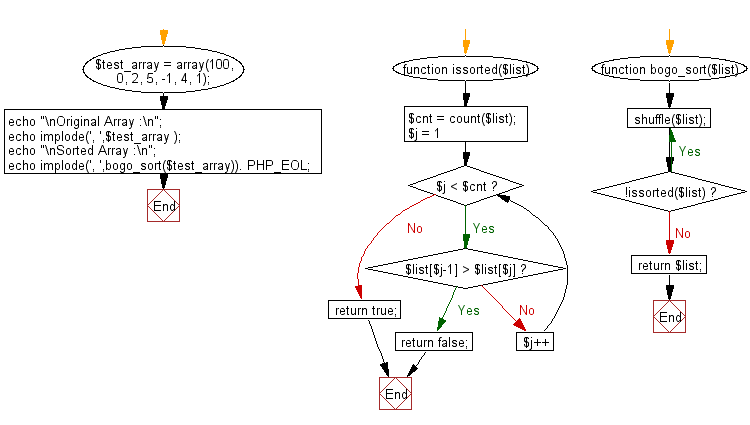﻿ PHP: Sort a list of elements using Bogo sort - w3resource

# PHP Searching and Sorting Algorithm: Bogo sort

## PHP Searching and Sorting Algorithm: Exercise-14 with Solution

Write a PHP program to sort a list of elements using Bogo sort.

In computer science, bogosort is a particularly ineffective sorting algorithm based on the generate and test paradigm. The algorithm successively generates permutations of its input until it finds one that is sorted. It is not useful for sorting but may be used for educational purposes, to contrast it with other more realistic algorithms.

Sample Solution :

PHP Code :

``````<?php
function issorted(\$list)
{
\$cnt = count(\$list);
for(\$j = 1; \$j < \$cnt; \$j++)
{
if(\$list[\$j-1] > \$list[\$j])
{
return false;
}
}
return true;
}
function bogo_sort(\$list)
{
do
{
shuffle(\$list);
}
while(!issorted(\$list));
return \$list;
}
\$test_array = array(100, 0, 2, 5, -1, 4, 1);
echo "\nOriginal Array :\n";
echo implode(', ',\$test_array );
echo "\nSorted Array :\n";
echo implode(', ',bogo_sort(\$test_array)). PHP_EOL;
?>
```
```

Sample Output:

```Original Array :
100, 0, 2, 5, -1, 4, 1
Sorted Array :
-1, 0, 1, 2, 4, 5, 100
```

Flowchart :PHP Code Editor:

Have another way to solve this solution? Contribute your code (and comments) through Disqus.

What is the difficulty level of this exercise?

Test your Programming skills with w3resource's quiz.

﻿